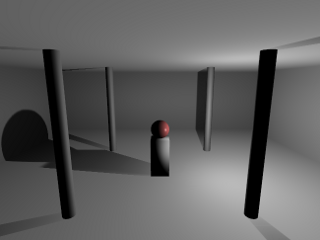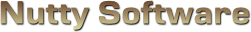Sorry, it appears you don't have support for WebGL.

Please note this demo uses large shaders, which can take some time to compile. Please allow a couple seconds for the demo to load.

In order to run this demo, you must meet the following requirements.

• You have a WebGL compatible video card with the latest drivers.
• Your video card is not blacklisted. You can check the current blacklist on Khronos.

Some browsers may require additional configuration in order to get WebGL to run. If you are having problems running this demo, visit the following sites.

#### Options

Animate Light
Spotlight
Point Light
							
/// <summary>
/// Vertex shader for rendering the depth values to a texture.
/// </summary>

/// <summary>
/// Attributes.
/// <summary>
attribute vec3 Vertex;

/// <summary>
/// Uniform variables.
/// <summary>
uniform mat4 ProjectionMatrix;
uniform mat4 ViewMatrix;
uniform mat4 ModelMatrix;
uniform vec3 ModelScale;

/// <summary>
/// Varying variables.
/// <summary>
varying vec4 vPosition;

/// <summary>
/// </summary>
void main ()
{
vPosition = ViewMatrix * ModelMatrix * vec4(Vertex * ModelScale, 1.0);
gl_Position = ProjectionMatrix * vPosition;
}


							
/// <summary>
/// Vertex shader for performing a seperable blur on the specified texture.
/// </summary>

/// <summary>
/// Attributes.
/// <summary>
attribute vec3 Vertex;
attribute vec2 Uv;

/// <summary>
/// Uniform variables.
/// <summary>
uniform mat4 ProjectionMatrix;

/// <summary>
/// Varying variables.
/// <summary>
varying vec2 vUv;

/// <summary>
/// <summary>
void main ()
{
gl_Position = ProjectionMatrix * vec4(Vertex, 1.0);
vUv = Uv;
}


							
/// <summary>
/// </summary>

/// <summary>
/// Material source structure.
/// <summary>
struct MaterialSource
{
vec3 Ambient;
vec4 Diffuse;
vec3 Specular;
float Shininess;
vec2 TextureOffset;
vec2 TextureScale;
};

/// <summary>
/// Attributes.
/// <summary>
attribute vec3 Vertex;
attribute vec2 Uv;
attribute vec3 Normal;

/// <summary>
/// Uniform variables.
/// <summary>
uniform mat4 ProjectionMatrix;
uniform mat4 ViewMatrix;
uniform mat4 ModelMatrix;
uniform vec3 ModelScale;
uniform mat4 LightSourceProjectionMatrix;
uniform mat4 LightSourceViewMatrix;

uniform int NumLight;
uniform MaterialSource Material;

/// <summary>
/// The scale matrix is used to push the projected vertex into the 0.0 - 1.0 region.
/// Similar in role to a * 0.5 + 0.5, where -1.0 < a < 1.0.
/// <summary>
const mat4 ScaleMatrix = mat4(0.5, 0.0, 0.0, 0.0, 0.0, 0.5, 0.0, 0.0, 0.0, 0.0, 0.5, 0.0, 0.5, 0.5, 0.5, 1.0);

/// <summary>
/// Varying variables.
/// <summary>
varying vec4 vWorldVertex;
varying vec3 vWorldNormal;
varying vec2 vUv;
varying vec3 vViewVec;
varying vec4 vPosition;

/// <summary>
/// <summary>
void main ()
{
// Standard basic lighting preperation
vWorldVertex = ModelMatrix * vec4(Vertex * ModelScale, 1.0);
vec4 viewVertex = ViewMatrix * vWorldVertex;
gl_Position = ProjectionMatrix * viewVertex;

vUv = Material.TextureOffset + (Uv * Material.TextureScale);

vWorldNormal = normalize(mat3(ModelMatrix) * Normal);

vViewVec = normalize(-viewVertex.xyz);

// Project the vertex from the light's point of view
vPosition = ScaleMatrix * LightSourceProjectionMatrix * LightSourceViewMatrix * vWorldVertex;
}


							
/// <summary>
/// Vertex shader for rendering the depth map to screen.
/// </summary>

/// <summary>
/// Attributes.
/// <summary>
attribute vec3 Vertex;
attribute vec2 Uv;

/// <summary>
/// Uniform variables.
/// <summary>
uniform mat4 ProjectionMatrix;

/// <summary>
/// Varying variables.
/// <summary>
varying vec2 vUv;

/// <summary>
/// <summary>
void main ()
{
gl_Position = ProjectionMatrix * vec4(Vertex, 1.0);
vUv = Uv;
}


							
/// <summary>
/// Fragment shader for rendering the depth values to a texture.
/// </summary>

#ifdef GL_ES
precision highp float;
#endif

/// <summary>
/// Linear depth calculation.
/// <summary>
const float Near = 1.0;
const float Far = 30.0;
const float LinearDepthConstant = 1.0 / (Far - Near);

/// <summary>
/// Specifies the type of shadow map filtering to perform.
/// 0 = None
/// 1 = PCM
/// 2 = VSM
/// 3 = ESM
///
/// VSM is treated differently as it must store both moments into the RGBA component.
/// </summary>
uniform int FilterType;

/// <summary>
/// Varying variables.
/// <summary>
varying vec4 vPosition;

/// <summary>
/// Pack a floating point value into an RGBA (32bpp).
/// Used by SSM, PCF, and ESM.
///
/// Note that video cards apply some sort of bias (error?) to pixels,
/// so we must correct for that by subtracting the next component's
/// value from the previous component.
/// </summary>
vec4 pack (float depth)
{
const vec4 bias = vec4(1.0 / 255.0,
1.0 / 255.0,
1.0 / 255.0,
0.0);

float r = depth;
float g = fract(r * 255.0);
float b = fract(g * 255.0);
float a = fract(b * 255.0);
vec4 colour = vec4(r, g, b, a);

return colour - (colour.yzww * bias);
}

/// <summary>
/// Pack a floating point value into a vec2 (16bpp).
/// Used by VSM.
/// </summary>
vec2 packHalf (float depth)
{
const vec2 bias = vec2(1.0 / 255.0,
0.0);

vec2 colour = vec2(depth, fract(depth * 255.0));
return colour - (colour.yy * bias);
}

/// <summary>
/// </summary>
void main ()
{
// Linear depth
float linearDepth = length(vPosition) * LinearDepthConstant;

if ( FilterType == 2 )
{
//
// Encode moments to RG/BA
//
//float moment1 = gl_FragCoord.z;
float moment1 = linearDepth;
float moment2 = moment1 * moment1;
gl_FragColor = vec4(packHalf(moment1), packHalf(moment2));
}
else
{
//
// Store screen-space z-coordinate or linear depth value (better precision)
//
//gl_FragColor = pack(gl_FragCoord.z);
gl_FragColor = pack(linearDepth);
}
}


							
/// <summary>
/// Fragment shader for performing a seperable blur on the specified texture.
/// </summary>

#ifdef GL_ES
precision highp float;
#endif

/// <summary>
/// Uniform variables.
/// <summary>
uniform vec2 TexelSize;
uniform sampler2D Sample0;

uniform int Orientation;
uniform int BlurAmount;

/// <summary>
/// Varying variables.
/// <summary>
varying vec2 vUv;

/// <summary>
/// Gets the Gaussian value in the first dimension.
/// </summary>
/// <param name="x">Distance from origin on the x-axis.</param>
/// <param name="deviation">Standard deviation.</param>
/// <returns>The gaussian value on the x-axis.</returns>
float Gaussian (float x, float deviation)
{
return (1.0 / sqrt(2.0 * 3.141592 * deviation)) * exp(-((x * x) / (2.0 * deviation)));
}

/// <summary>
/// <summary>
void main ()
{
float halfBlur = float(BlurAmount) * 0.5;
//float deviation = halfBlur * 0.5;
vec4 colour;

if ( Orientation == 0 )
{
// Blur horizontal
for (int i = 0; i < 10; ++i)
{
if ( i >= BlurAmount )
break;

float offset = float(i) - halfBlur;
colour += texture2D(Sample0, vUv + vec2(offset * TexelSize.x, 0.0)) /* Gaussian(offset, deviation)*/;
}
}
else
{
// Blur vertical
for (int i = 0; i < 10; ++i)
{
if ( i >= BlurAmount )
break;

float offset = float(i) - halfBlur;
colour += texture2D(Sample0, vUv + vec2(0.0, offset * TexelSize.y)) /* Gaussian(offset, deviation)*/;
}
}

// Calculate average
colour = colour / float(BlurAmount);

// Apply colour
gl_FragColor = colour;
}


							
/// <summary>
/// </summary>

#ifdef GL_ES
precision highp float;
#endif

/// <summary>
/// Linear depth calculation.
/// <summary>
const float Near = 1.0;
const float Far = 30.0;
const float LinearDepthConstant = 1.0 / (Far - Near);

/// <summary>
/// Light source structure.
/// <summary>
struct LightSource
{
int Type;
vec3 Position;
vec3 Attenuation;
vec3 Direction;
vec3 Colour;
float OuterCutoff;
float InnerCutoff;
float Exponent;
};

/// <summary>
/// Material source structure.
/// <summary>
struct MaterialSource
{
vec3 Ambient;
vec4 Diffuse;
vec3 Specular;
float Shininess;
vec2 TextureOffset;
vec2 TextureScale;
};

/// <summary>
/// Uniform variables.
/// <summary>
uniform int NumLight;
uniform LightSource Light;
uniform MaterialSource Material;
uniform sampler2D DepthMap;
uniform int FilterType;

/// <summary>
/// Varying variables.
/// <summary>
varying vec4 vWorldVertex;
varying vec3 vWorldNormal;
varying vec2 vUv;
varying vec3 vViewVec;
varying vec4 vPosition;

/// <summary>
/// Unpack an RGBA pixel to floating point value.
/// </summary>
float unpack (vec4 colour)
{
const vec4 bitShifts = vec4(1.0,
1.0 / 255.0,
1.0 / (255.0 * 255.0),
1.0 / (255.0 * 255.0 * 255.0));
return dot(colour, bitShifts);
}

/// <summary>
/// Unpack a vec2 to a floating point (used by VSM).
/// </summary>
float unpackHalf (vec2 colour)
{
return colour.x + (colour.y / 255.0);
}

/// <summary>
/// Calculate Chebychev's inequality.
/// <summary>
/// <param name="moments">
/// moments.x = mean
/// moments.y = mean^2
/// </param>
/// <param name="t">Current depth value.</param>
/// <returns>The upper bound (0.0, 1.0), or rather the amount to shadow the current fragment colour.</param>
float ChebychevInequality (vec2 moments, float t)
{
// No shadow if depth of fragment is in front
if ( t <= moments.x )
return 1.0;

// Calculate variance, which is actually the amount of
// error due to precision loss from fp32 to RG/BA
// (moment1 / moment2)
float variance = moments.y - (moments.x * moments.x);
variance = max(variance, 0.02);

// Calculate the upper bound
float d = t - moments.x;
return variance / (variance + d * d);
}

/// <summary>
/// VSM can suffer from light bleeding when shadows overlap. This method
/// tweaks the chebychev upper bound to eliminate the bleeding, but at the
/// expense of creating a shadow with sharper, darker edges.
/// <summary>
float VsmFixLightBleed (float pMax, float amount)
{
return clamp((pMax - amount) / (1.0 - amount), 0.0, 1.0);
}

/// <summary>
/// <summary>
void main ()
{
// vWorldNormal is interpolated when passed into the fragment shader.
// We need to renormalize the vector so that it stays at unit length.
vec3 normal = normalize(vWorldNormal);

// Colour the fragment as normal
vec3 colour = Material.Ambient;
for (int i = 0; i < 4; ++i)
{
if ( i >= NumLight )
break;

// Calculate diffuse term
vec3 lightVec = normalize(Light[i].Position - vWorldVertex.xyz);
float l = dot(normal, lightVec);
if ( l > 0.0 )
{
// Calculate spotlight effect
float spotlight = 1.0;
if ( Light[i].Type == 1 )
{
spotlight = max(-dot(lightVec, Light[i].Direction), 0.0);
float spotlightFade = clamp((Light[i].OuterCutoff - spotlight) / (Light[i].OuterCutoff - Light[i].InnerCutoff), 0.0, 1.0);
spotlight = pow(spotlight * spotlightFade, Light[i].Exponent);
}

// Calculate specular term
vec3 r = -normalize(reflect(lightVec, normal));
float s = pow(max(dot(r, vViewVec), 0.0), Material.Shininess);

// Calculate attenuation factor
float d = distance(vWorldVertex.xyz, Light[i].Position);
float a = 1.0 / (Light[i].Attenuation.x + (Light[i].Attenuation.y * d) + (Light[i].Attenuation.z * d * d));

colour += ((Material.Diffuse.xyz * l) + (Material.Specular * s)) * Light[i].Colour * a * spotlight;
}
}

//
//
vec3 depth = vPosition.xyz / vPosition.w;
depth.z = length(vWorldVertex.xyz - Light.Position) * LinearDepthConstant;
if ( FilterType == 0 )
{
//
// No filtering, just render the shadow map
//

// Offset depth a bit
// This causes "Peter Panning", but solves "Shadow Acne"
depth.z *= 0.96;

if ( depth.z > shadowDepth )
}
else if ( FilterType == 1 )
{
//
// Percentage closer algorithm
// ie: Just sample nearby fragments
//

// Offset depth a bit
// This causes "Peter Panning", but solves "Shadow Acne"
depth.z *= 0.96;

float texelSize = 1.0 / 512.0;
for (int y = -1; y <= 1; ++y)
{
for (int x = -1; x <= 1; ++x)
{
vec2 offset = depth.xy + vec2(float(x) * texelSize, float(y) * texelSize);
if ( (offset.x >= 0.0) && (offset.x <= 1.0) && (offset.y >= 0.0) && (offset.y <= 1.0) )
{
// Decode from RGBA to float
if ( depth.z > shadowDepth )
}
}
}
}
else if ( FilterType == 2 )
{
//
//
vec4 texel = texture2D(DepthMap, depth.xy);
vec2 moments = vec2(unpackHalf(texel.xy), unpackHalf(texel.zw));
}
else
{
//
//
float c = 4.0;
vec4 texel = texture2D(DepthMap, depth.xy);
shadow = clamp(exp(-c * (depth.z - unpack(texel))), 0.0, 1.0);
}

//
//
gl_FragColor = clamp(vec4(colour * shadow, Material.Diffuse.w), 0.0, 1.0);
}


							
/// <summary>
/// Fragment shader for rendering the depth map to screen.
/// </summary>

#ifdef GL_ES
precision highp float;
#endif

/// <summary>
/// Uniform variables.
/// <summary>
uniform int FilterType;
uniform sampler2D Sample0;

/// <summary>
/// Varying variables.
/// <summary>
varying vec2 vUv;

/// <summary>
/// Unpack an RGBA pixel to floating point value.
/// </summary>
float unpack (vec4 colour)
{
const vec4 bitShifts = vec4(1.0,
1.0 / 255.0,
1.0 / (255.0 * 255.0),
1.0 / (255.0 * 255.0 * 255.0));
return dot(colour, bitShifts);
}

/// <summary>
/// Unpack a vec2 to a floating point (used by VSM).
/// </summary>
float unpackHalf (vec2 colour)
{
return colour.x + (colour.y / 255.0);
}

/// <summary>
/// <summary>
void main ()
{
float depth = 0.0;
if ( FilterType == 2 )
depth = unpackHalf(texture2D(Sample0, vUv).xy);
else
depth = unpack(texture2D(Sample0, vUv));

gl_FragColor = vec4(depth, depth, depth, 1.0);
}



Shadow mapping is a hardware accelerated technique for casting shadows in a 3D scene. It has become the industry standard method for casting shadows due to its simplicity, its rendering speed, and its ability to produce soft shadows. This article will go over the shadow mapping technique as well as several filtering algorithms that are demonstrated in the interactive WebGL demo.

## The Basics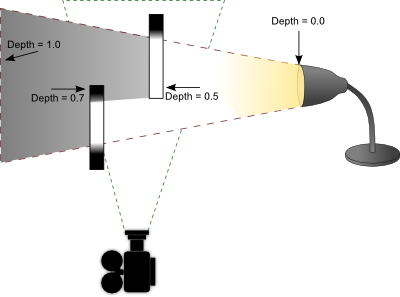Shadow mapping has a very basic process. The idea is to render the scene from the light's point of view and record the distance between the vertex and the light source in a texture object. This texture is referred to as a depth map. Objects closer to the light source will have a small depth value (closer to 0.0) and objects furthest from the light source will have a larger depth value (closer to 1.0). There are two ways to record depth values. One is to use the projected z-coordinate (non-linear) and the other is to use linear depth. Both of these processes are described later. Once you generate this depth map, you then render your scene as you normally would from your camera's point of view. In your fragment shader, you perform lighting calculations as you normally would. As a final step, you determine if that fragment is in shadow by projecting its vertex from the light's point of view. If the distance between that vertex and the light source is greater than what is recorded in the depth map, then that fragment must be in shadow because something closer to the light source is occluding it. If the distance is less than what is recorded in the depth map, then that fragment is not in shadow and is probably casting its own shadow elsewhere in the scene. The stages are illustrated below.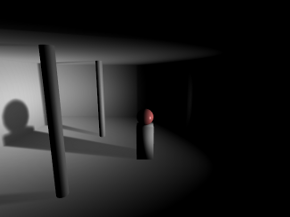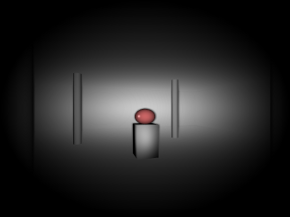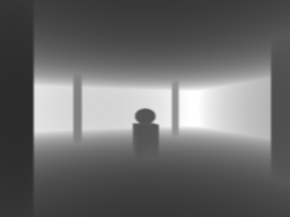Left: As seen from the camera.

Middle: As seen from the light source.

Right: Depth as seen from the light source.

## Getting Started

There's a couple caveats you need to be aware of before getting started with shadow mapping. Firstly, OpenGL ES 2.0 (WebGL) does not support the depth component texture format. In other words, you cannot render the depth values directly to texture. You must calculate this yourself and render the result to the colour buffer. This is not necessarily a bad thing. Traditionally the z-coordinate from a projected vertex was used for depth map comparisons in shadow mapping. The problem however is there is a loss of precision with this method, leading to something called "shadow acne".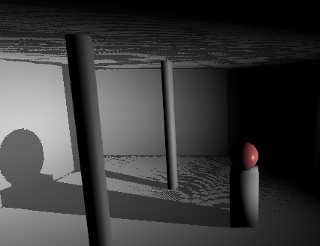Shadow acne is what happens when you perform comparisons with floating point values that are neck and neck with each other, which is a general problem in all forms of digital computing. What happens is the rounding error causes the shadow test to pass sometimes and fail other times, creating random spots of false shadows in your scene. One way to combat this issue is to apply a small offset to your polygons when rendering the depth map or a small offset to your shadow map depth calculations; however this can lead into another problem called "peter panning".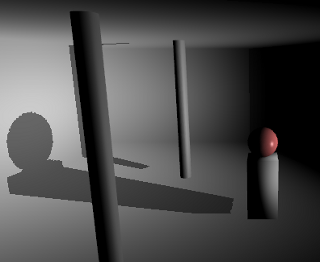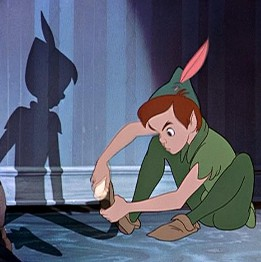Image of Peter Pan and his shadow. From Walt Disney's "Peter Pan", 1953.

The term "Peter Panning" came from the fictional character Peter Pan, whose shadow could detach from Peter and either assist or jest with him at times. As shown from the screenshot above, shadow acne has been removed at the expense of shadows appearing disconnected from their sources due to using a large polygon offset.

One way to minimize both shadow acne and peter panning is to use linear depth. What this means is that instead of using the projected Z-coordinate, we instead calculate the distance between the vertex and the light source in view space. You still need to map the result between 0.0 and 1.0, so you would divide the value by the maximum distance a vertex can have from its light source (ie: the far clipping plane). You use this same divisor later in the shadow test to determine if a vertex is inside or outside of a shadow. The result is a depth value with much better and equal precision throughout the viewing frustum. It doesn't outright eliminate shadow acne, but it helps. When combined with filtering algorithms such as VSM or ESM (explained later), it's virtually a non-issue.

## Rendering the Depth Map

OpenGL ES 2.0 (WebGL) does not support the depth component texture format. We need to render the depth values into the RGBA fragment. This is performed in the depth.vs and depth.fs shaders. You want to pass in the light source projection and view matrices. If you're using perspective projection, you should use an aspect ratio of 1.0 and an FOV of 90 degrees. This will produce an even square with good viewing coverage. You can optionally use an orthographic projection for directional light sources like the sun. In the fragment shader, after you compute the distance and divide it by the far clipping plane, you need to store the floating point value into a 4x4 byte fragment. How do you do this? You use a carry-forward approach.

Example

Depth value = 0.784653

R = 0.784653 * 255 = 200.086515 = 200 (carry fraction over)

G = 0.086515 * 255 = 22.061325 = 22

B = 0.061325 * 255 = 15.637875 = 15

A = 0.637875 * 255 = 162.658125 = 162

The depth value 0.784653 is stored in an RGBA fragment with the values (200, 22, 15, 162).

When you need to retrieve the depth value, you simply reverse the operations. This is done as follows.

Depth = (R / 255) + (G / 255$^2$) + (B / 255$^3$) + (A / 255$^4$)

Depth = (200 / 255) + (22 / 65025) + (15 / 16581375) + (162 / 4.2x10$^9$)

Depth = 0.784313 + 0.000338 + 0 + 0

Depth = 0.784651

You can see from the original depth value and the unpacked depth value that there is an error of 0.000002, which is quite acceptable. You'll also note that the green and alpha channels have a huge divisor, which makes them practically irrelevant in restoring a floating point value. As such, you should expect to get at least 16 bit precision and up to at most 24 bit precision using this method.

You can find the code for packing a floating point value into an RGBA vector in the depth.fs shader. There is however one more process involved not outlined in the math above. GPUs have some sort of floating point precision or bias issue with the depth values you store in the pixel. While it's not documented anywhere, the consensus is to subtract the next component's value from the previous component to correct the issue. That is, R -= G / 255, G -= B / 255, and B -= A / 255. You will notice this being performed in the depth fragment shader.

Once you have your depth map, you need to render your scene from the camera and check if each fragment is in shadow or not by comparing its vertex depth value projected by the light source with the depth value stored in the depth map. In the fragment shader shadowmap.fs, you will see these comparisons at the bottom of the main function.

In order to know what pixel to sample in the depth map, you need to project your vertex using the light's projection and view matrix.

$V_L = M_S * M_P * M_V * M_M * V$

Where

$M_S$ is a special scale matrix (or viewport matrix) to offset the vertex into the range 0.0 to 1.0.

$M_P$ is the light source projection matrix.

$M_V$ is the light source view matrix.

$M_M$ is the modelview matrix.

$V$ is the vertex being transformed.

$V_L$ is the projected vertex from the light source.

$V_L.xy$ contains the UV coordinates you can use to sample from the depth map. If uv is less than 0 or greater than 1, then this vertex is outside of the depth map bounds. This is possible if you didn't cover enough ground with your depth map. In this case, it's best not to check for any shadow and move on. $V_L.z / V_L.w$ would be your projected z-coordinate in the range 0.0 to 1.0 if inside the near - far clipping planes; otherwise < 0 or > 1 if outside. If you are using linear depth, this value is irrelevant. You would instead calculate the distance between the light source and your view space vertex and divide by the (far - near) clipping planes to force it within the range 0.0 to 1.0. All that remains is to compare this value against the depth map.

	
if ( depth > rgba2float(texture2D(DepthMap, VL.xy)) )
{
colour *= 0.5;
}
else
{
// Pixel not in shadow, do nothing
}



## Filtering

The problem with standard shadow mapping is the high amount of aliasing along the edges of the shadow. You also cannot take advantage of hardware blurring and mipmapping to produce smoother looking shadows. To get around these issues, several filtering algorithms are discussed below.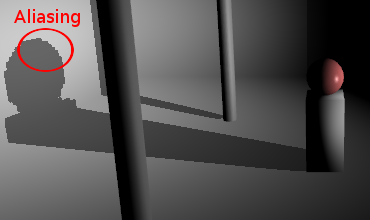## PCF

Percentage closer filtering is one of the first filtering algorithms invented and it works by adding an additional process into the standard shadow mapping technique. It attempts to smooth shadows by analyzing the shadow contributions from neighbouring pixels.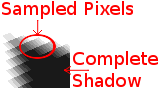The above example shows a 5x5 PCF filter. It doesn't use bilinear filtering so you can see how each neighbouring pixel is sampled. PCF can't operate on a blurred depth map. It requires an expensive 5x5 (or whatever kernel you wish to use) blurring operation for each fragment. While it's not recommended to use PCF, it does have the advantage of maintaining accuracy. It doesn't blur and thus "fudge" the depth map in order to get smoother shadows. With VSM and ESM, it's possible that blurring the depth map can produce false shadows, particularly along corners. It's a small tradeoff for an increase in speed.

## VSM and ESM

Variance Shadow Maps and Exponential Shadow Maps were designed to eliminate the performance penalty involved in smoothing shadows using the PCF algorithm. In particular, they didn't want to perform blurring during the render stage. They wanted to take advantage of the separable blur algorithm, as well as anti-aliasing and mipmaps/anisotropic filtering. The example below demonstrates the results achieved by blurring the depth map and using one of the aforementioned filtering algorithms.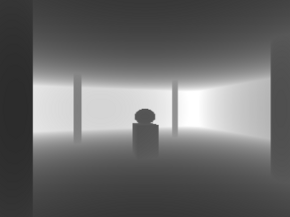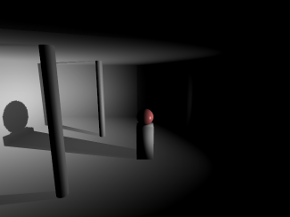No blurring (standard shadow map look and feel).3x3 blurring.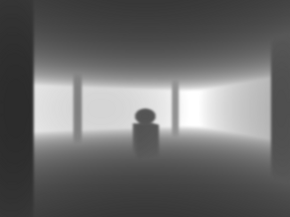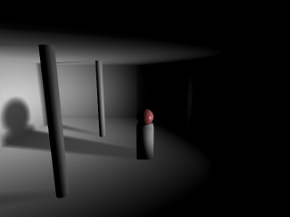5x5 blurring.

The separable blurring technique (also known as the box-blur) gets its name from the way it performs blurring. In the traditional sense, blurring is performed using a convolution filter. That is, an N x M matrix that samples neighbouring pixels and finds an average. A faster way to perform this activity is to separate the blurring into two passes. The first pass will blur all pixels horizontally. The second pass will blur all pixels vertically. The result is the same as performing blur with a convolution filter at a significantly faster speed.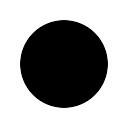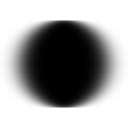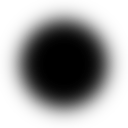Left: Unfiltered image.

Middle: Pass 1, horizontal blurring applied.

Right: Pass 2, vertical blurring applied.

What's unique here is that the lower resolution depth map you use, the more effective the blurring. A 256x256 depth map for instance can produce a very nice penumbra, whereas a 1024x1024 depth map requires a large kernel to blur it sufficiently enough. You have to find the right balance between resolution and blurring. To much of either can hinder performance.

VSM and ESM are virtually identical in terms of output quality, but there is one significant difference between the two. VSM requires you store both the depth and the depth squared in the depth map. This requires a 64 bit texture, which is not available on older hardware or even within OpenGL ES 2.0 (WebGL). As such, you need to compute and store both values as 16 bit into the RG and BA channels of the pixel. While the precision loss isn't that bad, a proper implementation would require more memory than what ESM requires.

The VSM formula is presented below.

S = ChebychevInequality(M1, M2, depth)

Where

$M1$ is the first moment from the depth map (= depth).

$M2$ is the second moment from the depth map (= depth * depth).

$depth$ is the light projected depth value of the current vertex.

$S$ is the computed shadow value, clamped to the range 0.0 and 1.0.

Chebychev's inequality function is what produces a gradient between 0.0 and 1.0 depending on whether or not the fragment is in shadow. The function is provided below.

	
float ChebychevInequality (vec2 moments, float t)
{
// No shadow if depth of fragment is in front
if ( t <= moments.x )
return 1.0;

// Calculate variance, which is actually the amount of
// error due to precision loss from fp32 to RG/BA
// (moment1 / moment2)
float variance = moments.y - (moments.x * moments.x);
variance = max(variance, 0.02);

// Calculate the upper bound
float d = t - moments.x;
return variance / (variance + d * d);
}



The maximum variance you see in this function is configurable. I chose a value of 0.02 because that worked well within the 16 bit precision error. Adjusting this value can have both a positive and negative effect on your shadows. If you find you are getting a lot of shadow acne, you need to raise the maximum value; otherwise you can lower it until such point where shadow acne is not apparent. Once you have your shadow value calculated from Chebychev's inequality function, you multiply the value against the current fragment colour. Pixels not in shadow will return a value of 1.0 and pixels within shadow will return a value less than 1.0.

An alternative to VSM is ESM. ESM is probably one of the best ways to currently filter shadow maps. It's memory efficient in that it only requires you store the depth value in the depth map and you can still take advantage of blurring the depth map, generate mipmaps, anisotropic filtering, etc. The ESM formula is presented below.

S = $e^{(-c * (d - z))}$

Where

$c$ is a constant value. Higher values produce darker shadows, lower values produce lighter shadows.

$d$ is the light projected depth value of the current vertex.

$z$ is the depth value stored in the depth map.

$S$ is the computed shadow value, clamped to the range 0.0 and 1.0.

Like VSM, the value returned from this function produces a gradient between 0.0 and 1.0. You take this value and multiply it against the current fragment colour. Pixels not in shadow will return a value of 1.0 and pixels within shadow will return a value less than 1.0.

## Point Lights

Point lights perform the exact same calculations as directional light sources, except you have to work with cubemaps. A point light may require up to six sides (left, front, right, back, top, and bottom) to receive shadows. This can add up to 6 times more calculations, which can have a significant impact on performance. If dealt with intelligently, you can deduce which sides of the cube are visible and perform only calculations on those faces. When possible, you should take advantage of multiple render targets to quickly produce depth cubemaps. OpenGL ES 2.0 (WebGL) unfortunately supports only one colour target, so that's not an option. Nevertheless, you should use point light shadows sparingly.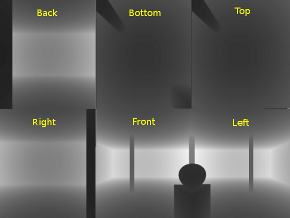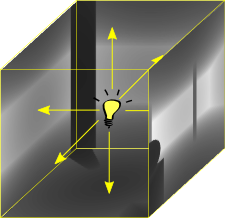The above screenshot shows the depth map for each side of the cubemap. When performing depth map comparisons, you need to find out which side in the cube you will be working with. To do this, calculate the vector from the light source to the vertex. This will point to the location in the cubemap containing the depth sample to compare against. The final result is a room with shadows emitted on all sides.